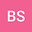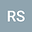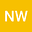Zero-Hopf Bifurcations and chaos of quadratic jerk systems
•••• Bo Sang,
• Rizgar Salih,
• Ning Wang
Bo Sang
Liaocheng university

Corresponding Author:[email protected]

Author ProfileRizgar Salih
University of Raparin
Author ProfileNing Wang
Tianjin University
Author Profile## Abstract

The purpose of this paper is to propose some coefficient conditions, characterizing the stability of periodic solutions bifurcated from zero-Hopf bifurcations of the general quadratic jerk system, and apply these theoretical results to a special jerk system in order to predict chaos. First, we characterize the zero-Hopf bifurcations of the general quadratic jerk system in $\mathbb{R}^3$. The coefficient conditions on stability of periodic solutions are obtained via the averaging theory of first order. Next, we apply the theoretical results to a two-parameter jerk system. Finally special attention is paid to a jerk system with one non-negative parameter $\epsilon$ and one non-linearity. By studying the continuation of periodic solution initiating at the zero-Hopf bifurcation, we numerically find a sequence of period doubling bifurcations which leads to the creation of chaotic attractor.